# Free Vibration of a Cantilever Beam(Continuous System)

4.3 Mathematical Analysis

For a cantilever beam subjected to free vibration, and the system is considered as continuous system in which the beam mass is considered as distributed along with the stiffness of the shaft, the equation of motion can be written as ( Meirovitch, 1967),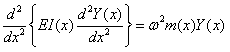(4.1)

Where, E is the modulus of rigidity of beam material, I is the moment of inertia of the beam cross-section, Y ( x ) is displacement in y direction at distance x from fixed end, ω  is the circular natural frequency, m is the mass per unit length, m = ρA(x)  ,  ρ is the material density,  x is the distance measured from the fixed end.

###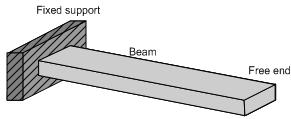Fig. 4.1 (a): A cantilever beam

###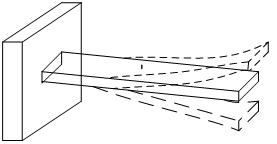Fig. 4.1 (b): The beam under free vibration

Fig. 4.1(a) shows of a cantilever beam with rectangular cross section, which can be subjected to bending vibration by giving a small initial displacement at the free end; and Fig. 4.1(b) depicts of cantilever beam under the free vibration.

We have following boundary conditions for a cantilever beam (Fig. 4.1)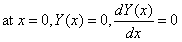(4.2)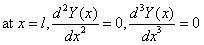(4.3)

For a uniform beam under free vibration from equation (4.1), we get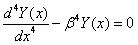(4.4)

with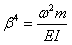The mode shapes for a continuous cantilever beam is given as

###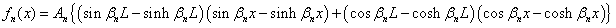(4.5)

Where

###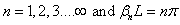A closed form of the circular natural frequency ω nf , from above equation of motion and boundary conditions can be written as,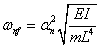(4.6)

Where

###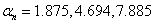So,

First natural frequency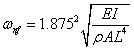(4.7)

Second natural frequency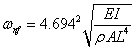(4.8)

Third natural frequency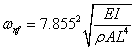(4.9)

The natural frequency is related with the circular natural frequency as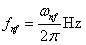(4.10)

where I , the moment of inertia of the beam cross-section, for a circular cross-section it is given as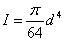(4.11)

Where, d is the diameter of cross section and for a rectangular cross section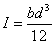(4.12)

Where b and d are the breadth and width of the beam cross-section as shown in the Fig. 4.2.

###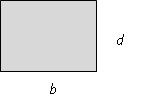Fig. 4.2: Cross-section of the cantilever beam

###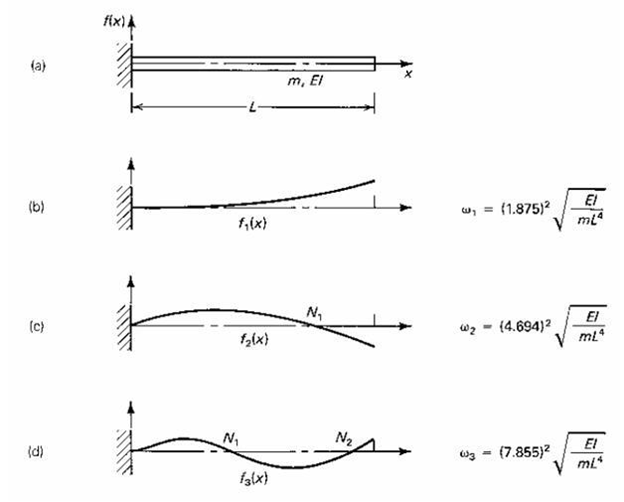Fig. 4.3: The first three undamped natural frequencies and mode shape of cantilever beam

Table 4.1 Material properties of various beams

 Material Density (kg/m 3 ) Young’s Modulus (N/m 2 ) Steel 7850 2.1×10 11 Copper 8933 1.2×10 11 Aluminum 2700 0.69×10 11

Table 4.2 Different geometries of the beam

 Length,L,(m) Breadth,b,(m Depth,h,(m) 0.45 0.02 0.003 0.65 0.04 0.003

Example 4.1: Obtain the undamped natural frequency of a steel beam with l = 0.45 m, d = 0.003 m, and b = 0.02 m.

First natural frequency

###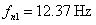Second natural frequency

###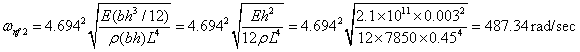###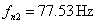Third natural frequency

###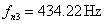The above frequencies have to be modified since there is a mass in the form of an accelerometer at the free end of the continuous beam. By continuous approach the solution is difficult since with tip mass the boundary condition at free end is now time dependent. Now we would explain a simpler procedure by which corrections to the natural frequency could be made so as to get closer to the measured natural frequency. In case of non-contacting sensors, there would not be any correction required since there will not be any additional tip mass on the beam.

4.3.1 Consideration of the mass of accelerometer onto a continuous cantilever beam

1. First natural frequency - Let us consider the beam specimen as mass-less with stiffness k and has a discrete effective mass, m eff , at the free end, which produces the same frequency as a continuous beam specimen without any tip mass. Hence, the natural frequency of discrete model of the beam without an accelerometer can be written as,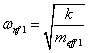(4.13)

with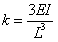(4.14)

From which the effective mass at tip can be written as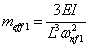(4.15)

with

###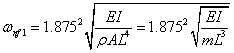or

###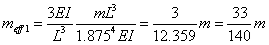Now, if we consider the mass of accelerometer, m acc , at the free end of the beam, than the total mass at free end will be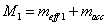(4.16)

So for the discrete beam with accelerometer, the theoretical fundamental natural frequency after considering the mass of accelerometer will be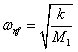(4.17)

Following the above procedure, other natural frequencies can be improved to account for the mass of the accelerometer. However, we need to obtain the effective stiffness of equivalent discrete system with additional supports at nodes (for the second natural frequency at one location and for the third natural frequency at two locations). The finite element method analysis is presented subsequently for the same.

Example 4.2   Obtain the fundamental natural frequency of beam by considering the mass of the sensor also.

Taking data from Tables 4.1 and 4.2 for steel,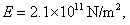###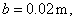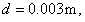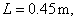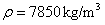,

###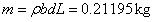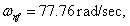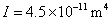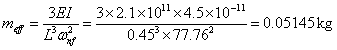The mass of accelerometer is 4.8 gm = 0.0048 kg, so the total mass will be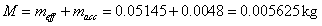Hence the corrected natural frequency after consideration of mass of the accelerometer would be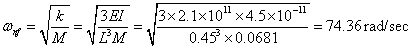which can be written as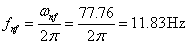2. Second natural frequency - Using FEM, we will find the second natural frequency of the cantilever beam (continuous system) having accelerometer mass at free end. The basic procedure is outlined here.

1.  In the first step, the geometry is divided into a number of small elements. The elements may be of different shapes and sizes.

2.  Then elemental equations are obtained for each element.

3.  In the third step the elemental equations are assembled to yield a system of global equations.

4.  Then assembled equations are modified by applying the prescribed boundary conditions.

5.  The last step is to find the solution from these modified equations.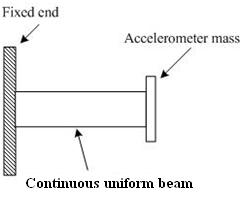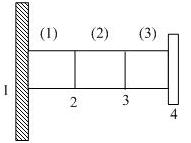Fig. 4.4 (a): A cantilever beam with a tip mass                 Fig. 4.4 (b): Disceretisation of the beam

into 3 elements

For a uniform beam, the elemental stiffness matrix (Tiwari, 2010; Dixit, 2009)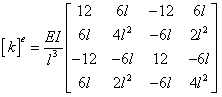(4.18)

and the consistence mass matrix is given as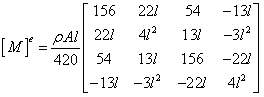(4.19)

1. First step: Divide the given beam geometry into three small elements as shown in Fig. 4.4 (b). In Fig. 4.4(b), numbers 1, 2, 3 and 4 represent the nodes.

2. Second step: Construct the elemental equations. For the first element, from equations (4.18) and (4.19), we have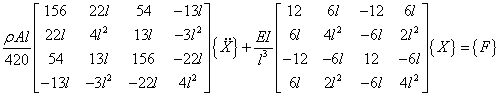(4.20)

Here { X } represents the vector of nodal variables (i.e. at the first and second nodes). In this case node variables are displacement and slope, the right hand side vector { F }, represents the force.

Then for the second element(4.21)

In this only nodal variables will change and for third element, the elemental equations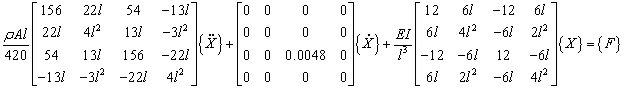(4.22)

Here, 0.0048 is the mass of the accelerometer in kg at free end of the cantilever beam.

3. Third step: Assemble all the elemental matrices to form a global matrix. The length of the each element  l = 0.45÷3 m and area is A = 0.002×0.03 m 2 , mass density of the beam material ρ = 7850 Kg/m 3 , and Young’s modulus of the beam E = 2.1 × 10 11 N/m . After substituting values of the l, ρ, d, E, A in elemental equations (4.20), (4.21) and (4.22); assembled equations become,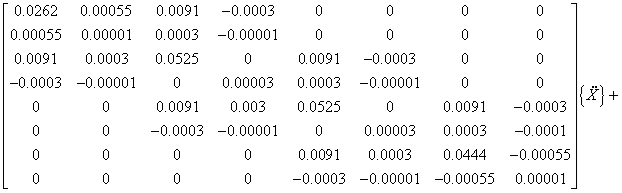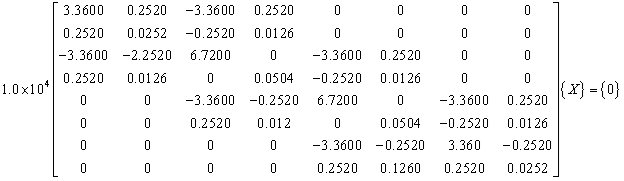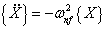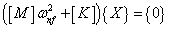(4.23)